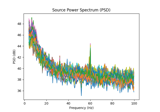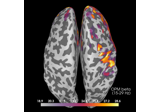# mne.minimum_norm.compute_source_psd#

mne.minimum_norm.compute_source_psd(raw, inverse_operator, lambda2=0.1111111111111111, method='dSPM', tmin=0.0, tmax=None, fmin=0.0, fmax=200.0, n_fft=2048, overlap=0.5, pick_ori=None, label=None, nave=1, pca=True, prepared=False, method_params=None, inv_split=None, bandwidth='hann', adaptive=False, low_bias=False, n_jobs=None, return_sensor=False, dB=False, *, verbose=None)[source]#

Compute source power spectral density (PSD).

Parameters
rawinstance of `Raw`

The raw data.

inverse_operatorinstance of `InverseOperator`

The inverse operator.

lambda2`float`

The regularization parameter.

method“MNE” | “dSPM” | “sLORETA”

Use minimum norm, dSPM (default), sLORETA, or eLORETA.

tmin`float`

The beginning of the time interval of interest (in seconds). Use 0. for the beginning of the file.

tmax

The end of the time interval of interest (in seconds). If None stop at the end of the file.

fmin`float`

The lower frequency of interest.

fmax`float`

The upper frequency of interest.

n_fft`int`

Window size for the FFT. Should be a power of 2.

overlap`float`

The overlap fraction between windows. Should be between 0 and 1. 0 means no overlap.

pick_ori`None` | “normal”

If “normal”, rather than pooling the orientations by taking the norm, only the radial component is kept. This is only implemented when working with loose orientations.

label`Label`

Restricts the source estimates to a given label.

nave`int`

The number of averages used to scale the noise covariance matrix.

pca`bool`

If True, the true dimension of data is estimated before running the time-frequency transforms. It reduces the computation times e.g. with a dataset that was maxfiltered (true dim is 64).

prepared`bool`

If True, do not call `prepare_inverse_operator()`.

method_params

Additional options for eLORETA. See Notes of `apply_inverse()`.

New in v0.16.

inv_split

Split inverse operator into inv_split parts in order to save memory.

New in v0.17.

bandwidth

The bandwidth of the multi taper windowing function in Hz. Can also be a string (e.g., ‘hann’) to use a single window.

For backward compatibility, the default is ‘hann’.

New in v0.17.

adaptive`bool`

Use adaptive weights to combine the tapered spectra into PSD (slow, use n_jobs >> 1 to speed up computation).

New in v0.17.

low_bias`bool`

Only use tapers with more than 90% spectral concentration within bandwidth.

New in v0.17.

n_jobs

The number of jobs to run in parallel. If `-1`, it is set to the number of CPU cores. Requires the `joblib` package. `None` (default) is a marker for ‘unset’ that will be interpreted as `n_jobs=1` (sequential execution) unless the call is performed under a `joblib.parallel_config` context manager that sets another value for `n_jobs`. It is only used if adaptive=True.

New in v0.17.

return_sensor`bool`

If True, return the sensor PSDs as an EvokedArray.

New in v0.17.

dB`bool`

If True (default False), return output it decibels.

New in v0.17.

verbose

Control verbosity of the logging output. If `None`, use the default verbosity level. See the logging documentation and `mne.verbose()` for details. Should only be passed as a keyword argument.

Returns
stc_psd

The PSD of each of the sources.

sensor_psdinstance of `EvokedArray`

The PSD of each sensor. Only returned if `return_sensor` is True.

Notes

Each window is multiplied by a window before processing, so using a non-zero overlap is recommended.

This function is different from `compute_source_psd_epochs()` in that:

1. `bandwidth='hann'` by default, skipping multitaper estimation

2. For convenience it wraps `mne.make_fixed_length_events()` and `mne.Epochs`.

Otherwise the two should produce identical results.

## Examples using `mne.minimum_norm.compute_source_psd`#Compute source power spectral density (PSD) in a label

Compute source power spectral density (PSD) in a labelCompute source power spectral density (PSD) of VectorView and OPM data

Compute source power spectral density (PSD) of VectorView and OPM data